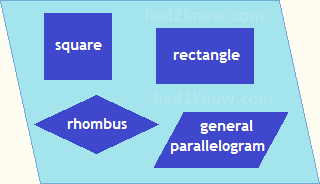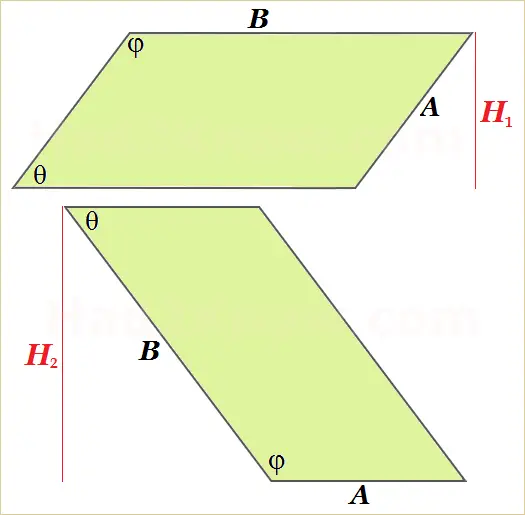# Parallelogram Calculator

 Parallelogram Calculator side lengths: A =     B = acute angle: θ = degrees Area = Short Height H1 = Long Height H2 = Short Diag. = Long Diag. =

In geometry, a parallelogram is a quadrilateral in which opposite sides are parallel and have equal length, and opposite angles have equal measure as well. The class of parallelograms includes rectangles and rhombuses (and of course squares, which are both rectangles and rhombuses).The acute and obtuse angles of a parallelogram always add up to 180 degrees. If you know the lengths of two adjacent sides and the value of the acute angle, you can compute the area, heights, and diagonals. The geometry formulas are explained below, and you can also use the calculator on the left.

The figure below shows the relations between the heights of the parallelogram and the sides. If A is the short side, B the long side, and θ the acute angle, then the short height, H1 is given by the formula

H1 = Asin(θ).

The long height is given by the formula

H2 = Bsin(θ).The area is given by several equivalent equations:

Area = BH1
= AH2
= ABsin(θ)
= H1H2/sin(θ)

The lengths of the diagonals can be computed with the formulas

Long Diag. = √A² + B² + 2ABcos(θ)

Short Diag. = √A² + B² - 2ABcos(θ)